Search IntMath
Close

450+ Math Lessons written by Math Professors and Teachers

5 Million+ Students Helped Each Year

1200+ Articles Written by Math Educators and Enthusiasts

Simplifying and Teaching Math for Over 23 Years

# How to draw y^2 = x - 2?

By Murray Bourne, 09 Apr 2009

Nuaja, a subscriber to the IntMath Newsletter, wrote recently:

How do I know how the graph should look like: For example: y2 = x - 2?

The first thing I recognize in that equation is the y2 term, which tells me it will be a parabola. (It won't be a circle, ellipse or hyperbola because there is an x term, but no x2 term. See Conic Sections.)

Let's start with the most basic parabola y = x2 and build up to the required answer.

## Example 1: y = x2

You could draw up a table and calculate the y-values for a set of x-values, like this:

 x y -4 -3 -2 -1 0 1 2 3 4 16 9 4 1 0 1 4 9 16

This gives us a series of points (-4,16), (-3,9), (-2,4) up to (4,16).

You then join these dots with a smooth curve and get something like the following.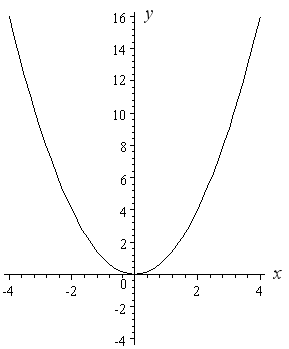Notice that the vertex of the parabola (the "pointy" end) is at the origin, (0, 0).

Now for all the curves that I draw below, I'm not going to draw up a table. It becomes tedious, and it can lead to incorrect graphs. It is better to be able to recognize the graph type (from the equation) and then know how to sketch it in the right place and with the right orientation.

I will consider the effect of small changes to the equation and then sketch my curve.

All of the following graphs have the same size and shape as the above curve. I am just moving that curve around to show you how it works.

## Example 2: y = x2 − 2

The only difference with the first graph that I drew (y = x2) and this one (y = x2 − 2) is the "minus 2". The "minus 2" means that all the y-values for the graph need to be moved down by 2 units.

So we just take our first curve and move it down 2 units. Our new curve's vertex is at −2 on the y-axis.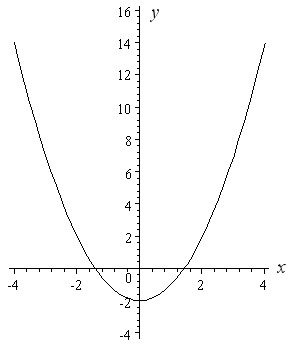Next, we see how to move the curve up (rather than down).

## Example 3: y = x2 + 3

The "plus 3" means we need to add 3 to all the y-values that we got for the basic curve y = x2. The resulting curve is 3 units higher than y = x2. Note that the vertex of the curve is at (0, 3) on the y-axis.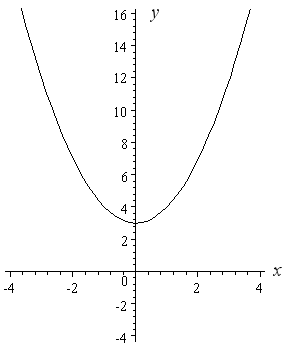Next we see how to move a curve left and right.

## Example 4: y = (x − 1)2

Note the brackets in this example - they make a big difference!

If we think about y = (x − 1)2 for a while, we realize the y-value will always be positive, except at x = 1 (where y will equal 0).

Before sketching, I will check another (easy) point to make sure I have the curve in the right place. Putting x = 0 is usually easy, so I substitute and get

y = (0 − 1)2

= 1

So the curve passes through (0, 1).

Here is the graph of y = (x − 1)2.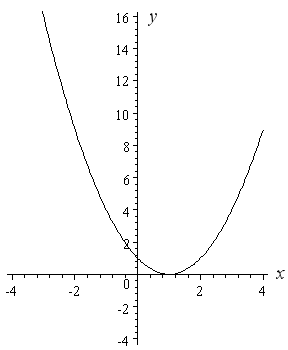## Example 5: y = (x + 2)2

With similar reasoning to the last example, I know that my curve is going to be completely above the x-axis, except at x = −2.

The "plus 2" in brackets has the effect of moving our parabola 2 units to the left.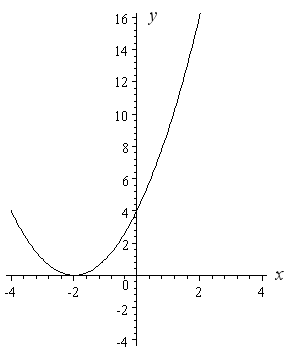## Rotating the Parabola

The original question from Anuja asked how to draw y2 = x − 4.

In this case, we don't have a simple y with an x2 term like all of the above examples. Now we have a situation where the parabola is rotated.

Let's go through the steps, starting with a basic rotated parabola.

## Example 6: y2 = x

The curve y2 = x represents a parabola rotated 90° to the right.

We actually have 2 functions,

y = √x (the top half of the parabola); and

y = −√x (the bottom half of the parabola)

Here is the curve y2 = x. It passes through (0, 0) and also (4,2) and (4,−2).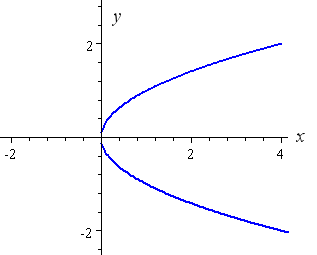[Notice that we get 2 values of y for each value of x larger than 0. This is not a function, it is called a relation.]

## Example 7: (y + 1)2 = x

If we think about the equation (y + 1)2 = x for a while, we can see that x will be positive for all values of y (since any value squared will be positive) except y = −1 (at which point x = 0).

In the equation (y + 1)2 = x, the "plus 1" in brackets has the effect of moving our rotated parabola down one unit.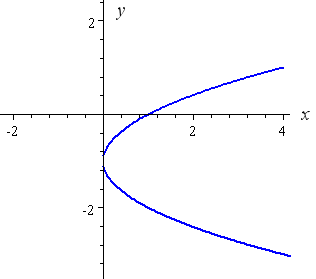## Example 8: (y − 3)2 = x

Using similar reasoning to the above example, the "minus 3" in brackets has the effect of moving the rotated parabola up 3 units.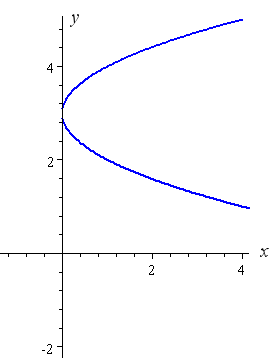## Example 9: y2 = x − 2

You can hopefully imagine what is going to happen now. We have a y2 term , so it means it will be a rotated parabola.

When x = 2, y = 0. The value of x cannot be less than 2, otherwise when we try to evaluate y we would be trying to find the square root of a negative number. Since out numbers are all real numbers, x must be greater than or equal to 2.

The "minus 2" term has the effect of shifting our parabola 2 units to the right.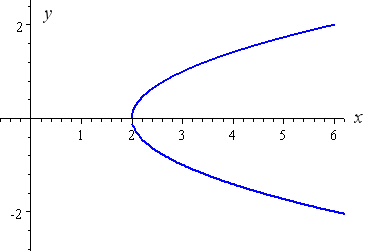I hope you can see now that if the equation was y2 = x + 2 (with a "plus"), then we would need to shift our rotated parabola to the left by 2 units.

Like all things, the best way how to learn graph sketching is through practice. Also, be observant and note the effect of plus, minus and brackets in each example.

### 72 Comments on “How to draw y^2 = x - 2?”

1. hassan says:

thanks, nice tip...

2. Nandan says:

Remarkable

3. MAVIS says:

I hv learned a lot, thanks. The problem with me is integration.

4. amane says:

5. kiran says:

thanks for help in maths nd i hav learned alot from this and my problem is planes

6. max says:

One word, FANTASTIC.

looking forward to the next e-mail.

7. hosea says:

Thanks for your explanation, it has assisted me much. Keep it up.

8. Munzir says:

thanks for the tip i nvr knew that sketching graphs are so easy and not so tedious. thank you very much

9. ricar says:

TNX A LOT! KEEP UP..

10. Masereka Hosea says:

11. Murray says:

Hi Masereka. Do you mean "Laplace"? You can find a chapter on it here: Laplace Transformation.

12. chirag hira says:

i do not understand how you drew the graph for y = x2( example 1)

can you please give me some pointers on how to go about doing these things

i am currently in grade 10 (std8)

plaese if any one can help

thanks alot

13. Murray says:

Hi Chirag. The table for example 1 is obtained by substituting the different x-values into y = x2. So for example, when x = -2, then y = (-2)2 = 4. This is graphed by putting a dot on the point (-2,4).

We then put dots for all the other points in the table (like (-4,16), then (-3,9) and so on. We join the dots with a smooth curve and end up with the parabola shown.

Hope that helps.

14. chirag hira says:

thanks alot zac that realy did help and made me think

thanks for all the help
chirag

15. Zach says:

I was wondering how to graph y^2=x in a graphing calculator

Thanks, Zach

16. Murray says:

Hi Zach

Since y^2 = x − 2 is a relation (has more than 1 y-value for each x-value) and not a function (which has a maximum of 1 y-value for each x-value), we need to split it into 2 separate functions and graph them together. So the first one will be y1 = √(x − 2) and the second one is y2 = −√(x − 2). When you graph these on the same axis, it will give the required result.

17. nimitz says:

thanks i know it

18. nandan says:

very good

19. Mike Theodore says:

Great examples.

20. peter says:

thanks so much this is so helpful!!

21. mohamed says:

it's easy to understand, sketching is so important so we need programm can draw the function in moments in this good and best website.

22. deedee says:

hey, thanks alot 4 d examples! it did help....
but i'm still confused on how d x and y values were derived from just an equation.
i hope u can pls shed more light on that...
thanks

23. Murray says:

@deedee: Are you referring to the table of values in Example 1?

They just come from substituting in values. The first one is x = -4, and substituting that into y = x^2 gives y = (-4)^2 = 16.

Hi,
I woul like to know how to find the simplest general formua of parabolic graph? and you can find the tangent line in it?

thank you.

25. Murray says:

Hi Shamsa. The simplest general formula for a parabola is given in the article: y = ax2 + bx + c.

You can find how to differentiate polynomials here: Derivatives of Polynomials.

Later on that page is an example of a tangent line.

26. jr says:

how do u find the parabola if all the numbers are positive?????

27. Murray says:

Hello jr. Do you mean if the question was y^2 = x + 2? Well, instead of moving the parabola to the right by 2 units (like I did at the end in the above example), you would move it to the left by 2 units, as mentioned below the final graph.

28. Caitlyn says:

How would you draw a graph if the question is only half a parabola?
y^2= √(-8x)

29. Murray says:

It's actually a "full" parabola, Caitlyn. The negative in front of the x just flips the parabola through the y-axis so the arms face to the left, instead of the right like the final examples above.

30. PdfoK FilE says:

Gret site. Thanks. I agree that this site is rather cognitive and useful especially for professionals.

31. Jovan says:

But how to draw a y=2-x^2..

32. Murray says:

Hi Jovan

Well, first can you sketch y = -x^2?

Then, what does the 2 out the front do?

33. tamirat tesfaye says:

How i can draw the graph of parabola when only two points are given.

34. Murray says:

@Tamarat: There are an infinite number of parabolas passing through 2 points. You always need 3 points to determine a parabola, and you also have to specify the direction of the axis (vertical, horizontal or at an angle).

35. gagangc says:

sir,
if i want to plot same function by finding intercepts and slopes,then y intercept will involve complex numbers. what it means?,cant i plot it if i take my y axis as imaginary axis?,in that case graph would be different.

36. Murray says:

@Gagangc: Interesting question! All the plots on this page assume real axes. You could, I guess, plot the ones involving complex numbers on the 3D complex plane, and the result would be something like the ones on this page: exponential 3D plots.

37. gagangc says:

Sir,

i tried sketching xy = 4 (hyperbola),using curve sketching by differentiation,i got x and y intercepts as infinity which i understood.but problem came when i tried finding maxima and minima as i had to equate (-4)/(x^2) to 0.what is it telling to me??i could not come to any conclusion.problem got bigger when i found second derivative.help me find solution.

38. Murray says:

@gagangc: A hyperbola in this orientation doesn't have a maximum or minimum! You'll find an example similar to your question on this page: Hyperbola.

39. gagangc says:

Sir,
If the real roots of a quadratic eqn are the x-intercepts, what do imaginary roots represent graphically?.(as in x^2+9).i am bit confused regarding this,help me to get out of this problem.

40. Murray says:

@gagangc: You can't represent complex roots on a real cartesian plane. However, we can plot complex numbers on the complex plane (with "reals" on the horizontal axis and "imaginary" on the vertical axis).

Finding these graph difficult to do. Could someone help me with sketch the graph of y squared = 25 - x squared I need to know how to work it out so we can apply it to other values. thanks.

42. Murray says:

Hi Brenda

43. Cheryl says:

how to sketch the graph of x=(y-2)^2 +4 ?? thank you 😀

44. Murray says:

Hello Cheryl. The answer for your question is contained in the article!

What orientation does it have? (Legs up? Right? Left? Down?)
What does the "-2" in the brackets do?
What does the "+4" do?

45. arthur says:

y=(x-2)*2

Great explanation thanks, it's good when you can visualize what is going on.

47. gummi says:

how do you do y = x2 + 3x +3

48. Murray says:

49. rahul gupta says:

how to draw the graph of |x|+|y|=1

50. Frank says:

You should teach my pre-calc class instead

51. Amrit Kohli says:

I want to know how you can define the parabolas :

zx = y^2
zy = x^2
zy = -x^2
zx = -y^2

as one unified equation? the property of a parabola that i am most interested in is how it approaches its respective axis asymptotically as z grows (or shrinks). how would one define these parabolas with one equation? is it even possible? does anyone know what i'm talking about?

for example, if zx = y^2 and zx = -y^2, how would one express that zx = y^2 and zx = -y^2 simultaneously?

52. Murray says:

Hello Amrit

Actually, these parabolas won't "approach any axis asymptotically". A parabola's axis is the line that cuts the parabola into 2 equal halves (so each parabola cuts its axis once only, at the vertex.)

Probably the best you can do in terms of expressing these as one equation is to settle for 2.

(zx)^2 = y^2 will give your 1st and 4th equations

(zy)^2 = x^2 will give your 2nd and 3rd equations

Or perhaps you could set it up as a linear sum:

a (zx)^2 + b (zy)^2 = a y^2 + b x^2

Then if a = 1 and b = 0, you have your 1st and 4th cases, and a = 0, b = 1 gives you the 2nd and 3rd cases.

Regards

53. Amrit Kohli says:

Hey Murray,

thanks for the insights! that helps me a ton.

what i meant by the parabola reaching its axis asymptotically, i should have been more clear. consider this equation:

x = - y^2/z

that's just one example of the four equations i was originally working with. this parabola opens along the negative x axis. so, consider what happens to the equation as z goes to infinity. what i was trying to say is that the parabola opens wider and wider until it is asymptotically approaching the y axis. it will eventually appear flat, but is really extending asymptotically along the y-axis.

does that make sense?

when you say "1st and 4th cases" and "3rd and 2nd cases," what exactly did you mean? won't a and b be any arbitrary number? so, aren't there an infinite number of cases?

what if a and b are fractions?

thanks for having the discussion and for getting me closer to what i'm trying to express, which is a unified equation of energy and mass. i'm trying to suggest that Einstein was wrong to include c^2 in his famous equation. these equations are essential to proving that. 🙂

54. Murray says:

Hi Amrit

I originally read your "zx" as zx, but now that I see you mean

z = y^2 / x,

it makes a lot more sense.

Indeed, there are asymptotes going on here.

The above graph looks like this: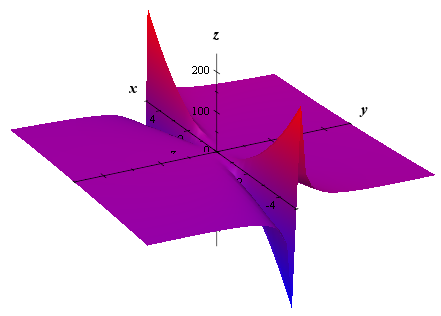There is a vertical "wall" that should not actually appear in the graph - it is actually the asymptote.

Here I've added z = x^2 / y: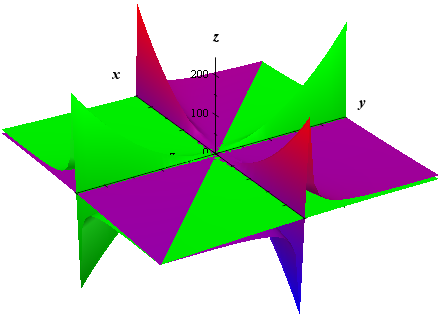Next, here are all 4 of your graphs: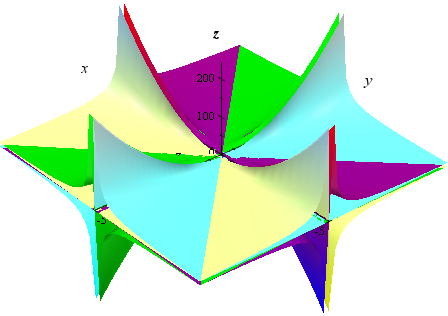I still don't believe it's possible to achieve one single equation.

This would give you 2 of them (your first and last):

z^2 = y^4 / x^2

And this would give you the other 2:

z^2 = x^4 / y^2

Regards

55. Amrit Kohli says:

Hi Murray,

z is actually a constant. how does that change things?

sorry i have been so unclear. this is a learning process for me because i have forgotten all my algebra. where i am going with all of this might have me take a derivative because i am actually looking for the equation that will give me the volume of the sphere that would sit on all these equations.

like, in your third drawing, imagine setting a sphere flush to the parabolas and now calculate its volume, or mass even, and that is the equation i am after.

it would have infinite volume and infinite mass because all these equations are asymptotically going "up" against their limits, right? so, am i really looking for the limit as what approaches what?

again, i am uncertain what i am looking for as of yet. but your expertise is very helpful to me. so, thank you!

amrit

56. Murray says:

Hi Amrit

In your first post, you said "as z grows (or shrinks)", and later you said "as z goes to infinity", which means z is a variable.

Perhaps you mean it is a "variable constant".

Either way, it may be good for you to think of this as a 3-dimensional problem.

Perhaps you could get GeoGebra and play with it. See what I wrote here (similar to your equations, but different):

GeoGebra now with 3D graphs

It's a good tool for exploring these concepts - you can do it in 2D or 3D.

Regards.

57. Christopher says:

how to graph the curve x^2+3y+4x+6=0? also the curve y^2=-x?
what is their points of intersection and the area? ^_^

58. Murray says:

@Christopher: For the first one, just solve it for y.

The second one can be found in the article above.

The points of intersection are found by solving the 2 equations simultaneously. See: Algebraic solution of non-linear systems.

The enclosed area is found using integration. See: Area between curves using integration

59. Pablo jeynes says:

Great site. These ideas of shifting a curve vertically and horizontally and rotating are really gregat ways to see and understand a whole bunch of equations very easily. It should be explored more in high school as early as possible imo .

Just wondering about the rotated parabola idea.
It may be a little more hard to see for some students but perhaps it is better viewed as a reflection through the diagonal line y=x.
These kind of reflections can actually be explored in a fun way using a small mirror.
The reflection in y=x is the general way to swop over the y and an x graphically when they appear in an equation or function. This corresponds to inverting the function.
For example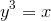and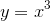are related by reflection in y=x and not by rotation. More spectacular is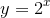and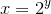the latter being a good introduction to logarithm function.
The rotation idea works for parabola because it is symmetric . However the left part of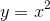transforms to the lower half of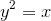rather than the upper part as might be envisaged using rotation.

60. Murray says:

@Pablo: Good suggestions! Thanks for your input.

61. Amrit Kohli says:

Hi Murray,

so, since my last post, i have been incubating on the ideas that you presented in your reply.

i have some more questions for you, if you would like to take a moment and consider them. i would certainly appreciate your help in thinking through this understanding i am formulating.

consider a sphere.

we know that the circumference of a circle over its diameter = π.

but how can you calculate the equivilent in 3D space, like for a sphere?

does the formula involve integrals? i am essentially trying to calculate the equation for the analogous concept of the circumference of a 2D circle on a 3D sphere. so, the "circumference" of a sphere is the surface area of the sphere, not just a 2D slice of the surface area. i'm not sure if the appropriate unit of this measurement is a square meter, or something else. it seems one could conceivably flatten a sphere by smashing it into a 2D mat, thereby driving its volume to zero, and then you'd have some kind of cracked and irregular shape that would not be easy to measure. you couldn't just take the "circumference" of the flattened "circle." it will still have a square surface area, wouldn't it? or is the unit cubic meters? i thought that was for volume.

anyway, i could google it, but the whole point of doing it this way, with a community, is to dissect the most primitive "givens" and understand each component of what we typically take for granted, what we take as simply "given," if you will 🙂

then, as you suspected, i would also like to calculate the "diameter" of a sphere. this appears to be the square sufrace area of the plane that bisects the sphere at its widest expanse, and i do not know how to calculate that either.

then, there is the equivilence to π that exists in the 2D realm as defined by circumference / diameter that i suspect also exists in the 3D realm when speaking of spheres.

so, what i'm asking is what does the "circumference" / "diameter" of a sphere equal? π? or some square or square root of π? or maybe an integral of π? well, wouldn't the integral of π be the volume of a sphere? hmmm.

anyway, this is where i am with my quest for the correct equation. i believe this is probably all Calculus II level math, probably even just Calculus I, but i have forgotten all my Calculus, and i get so much more than just the answers from you. i get a very unique and elemental perspective on my curiousity from your responses. and i don't know how i found this Blog, but if you help me with this, and i complete the formula i am attempting to work out, i am planning to post it here.

can you help me figure out how π works in 3D space?

and in return, i'll give you and your audience here The Theory of Everything.

i'm not kidding. i think it's worth it for you and your readers to consider it. just help me figure this part out with the sphere, and i'll show my full hand. it could very well be the big break we've been looking for...or, it could just be the musings of a lay engineer and carry no unique significance. but i'm willing to bet it's at least, AT LEAST, profound 🙂

looking forward to hearing back from you!

amrit

62. Hasnain says:

Sir, plz tell me that how to sketch a graph of y=under root, x+2

63. Murray says:

Hello Hasnain

Do you mean y = 1/ sqrt(x+2) ?

If so, What values of x are you allowed to use? which one(s) are not allowed?

What will happen as x gets very large?

What happens when x is very small (heading off in the negative direction?)

64. george lopez says:

what about when x is negative, per say, y=-x squared

65. Murray says:

@George: A minus in front of the x2 term results in a reflection in the x-axis. See How to reflect a graph through the x-axis, y-axis or Origin? for more information.

66. Rohini says:

How to draw graph of y^2=x^2(1-x)
How to explain it

67. Murray says:

@Rohini: I suggest you think of it as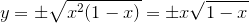Then graph each branch separately.

68. Anushka Maheshwary says:

Can you tell me how to draw the graph for y=√(1-x)?

69. Murray says:

@Anushka: Example 6 above and Example 1a on the page Domain and Range of a Function are related to your question. Do you think you can make the appropriate adjustments?

70. Robert S. says:

What if squares and square routes always mamtained their polarity? In other words; the square of -9 was -81 or the square route of -9 was -3. You could graph this and it wouldn't need an imaginary number like I. In reality I believe this should be true because squares and square routes seem of little use unless they refer to 0. I'm other words -3 x 3 is -9. So why do we need 3squared? Ur friend bob

71. mitchie says:

how do I draw the graph of y^2=4-x

72. Murray says:

@Mitchie: To start you off, what do you think y^2 = -x (without the 4) will look like?

### Comment Preview

HTML: You can use simple tags like <b>, <a href="...">, etc.

To enter math, you can can either:

1. Use simple calculator-like input in the following format (surround your math in backticks, or qq on tablet or phone):
a^2 = sqrt(b^2 + c^2)
(See more on ASCIIMath syntax); or
2. Use simple LaTeX in the following format. Surround your math with $$ and $$.
$$\int g dx = \sqrt{\frac{a}{b}}$$
(This is standard simple LaTeX.)

NOTE: You can mix both types of math entry in your comment.